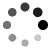[原创]2015年蓝桥杯省赛B组C/C++（试题+答案）前几题

2016-03-01 20:57:20 Tabris_ 阅读数：3614

https://blog.csdn.net/qq_33184171/article/details/50775612

89999=52488

## `12345678910111213141516171819``# include <stdio.h>int sum;void dfs(int n){ if(n>6) return ; if(n==6) sum++; for(int i=0;i<10;i++) { if(n==1&&i==0) continue; if(i==4) continue; dfs(n+1); }}int main(){ dfs(1); printf("\n%d\n",sum);}`

• ``````  ![这里写图片描述](http://img.blog.csdn.net/20160301180449804)
``````

1，因为是加法算式三是进位出来的 所以是三是1
2，因为三是1且三加祥能进位，只有1+9能进位，所以祥是9
**但是考虑可能有进位的情况祥可能为8或9 ***
⑴，当祥为8时8+1+1（进位的1）=10 这时羊只能为0 而0+任何1~9数均<10 不可能进位 ——不成立
⑵，当祥为9时9+1+1（进位的1）=1，羊为1 因为三为1 所以羊不为1 ——不成立

3，瑞+0=生只能是后面进位了，得到瑞+1等于生。

（到这可能有些不明白，解释下，这又是因为后位进1导致1+献=0 应为 1+献+1（进位的1）=0 即献=8）x=2时 x+y>=10，y>=8 而8,9已经存在—— 不成立
x=3时 x+y>=10，y>=7 8,9已经存在 也只能为7，但 x+y=3+7+10 z应为0 因0已经存在—— 不成立
x=4时 x+y>=10，y>=6 8,9已经存在 y可为6,7 当为6时z=0—— 不成立 当为7时 z=1 不成立
x=5时 x+y>=10，y>=5 8,9已经存在 y为7时 z为2 此时总式成立

***此题目用推理的方式做出来 上述只是他的证明过程 所以文字多 篇幅长 但是这都是思考出来的 在实际操作中很快就会得出结果 比写暴力的代码要省时的多 毕竟代码那么多的for语句 还有那么多数字加在一起 很容易出错了 虽然不用代码 有点违背蓝桥杯是C++比赛的事实 但是做出题来得分 取得名次 才是王道 ***

---------------------------------Update-----------------------------

7题：牌型种数